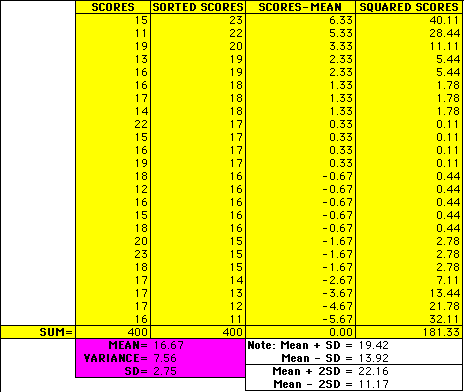# Is There a Standard for All This Deviation?

As useful as variance is as a measure, it poses a slight problem. When you are using variance you are dealing with squares of numbers. That makes it a little hard to relate the variance to the original data. So, how can you "undo" the squaring that took place earlier?

The way most statisticians choose to do this is by taking the square root of the variance. When this brilliant maneuver was made the great statistics gods named the new measurement standard deviation and it was good and the name stuck.

With this new measurement, the information derived through the variance can much more easily be applied to the original data. Look at your dice game data set again. Find the variance and take the square root to get the standard deviation.If you will add one standard deviation to your mean and subtract one standard deviation from your mean, you should find that a majority of your scores fall between those two numbers. If you add and subtract 2 standard deviations you should find that nearly all of your scores will fall between those two numbers.

Statisticians have found this fact to be very useful and in many cases they use this property to determine the probability of a given data point occurring. This allows them to determine the verity or falseness of hypotheses.

Now, you may see what would happen if you had chosen to use the absolute value of your deviation scores. If, however, you have already taken that path, you may now go to the end.